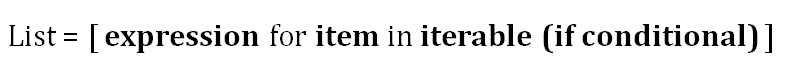1634123880

# How to Convert Tuple To List in Python with Example Code

Learn How to Convert Tuple To List in Python with Example Code

Use the built-in list() function to convert a tuple to a list in Python. Simply passes the given tuple as an argument to the function.

## Buddha Community1619518440

## top 30 Python Tips and Tricks for Beginners

Welcome to my Blog , In this article, you are going to learn the top 10 python tips and tricks.

### 8) Check The Memory Usage Of An Object.

#python #python hacks tricks #python learning tips #python programming tricks #python tips #python tips and tricks #python tips and tricks advanced #python tips and tricks for beginners #python tips tricks and techniques #python tutorial #tips and tricks in python #tips to learn python #top 30 python tips and tricks for beginners1619510796

## Lambda, Map, Filter functions in python

Welcome to my Blog, In this article, we will learn python lambda function, Map function, and filter function.

Lambda function in python: Lambda is a one line anonymous function and lambda takes any number of arguments but can only have one expression and python lambda syntax is

Syntax: x = lambda arguments : expression

Now i will show you some python lambda function examples:

#python #anonymous function python #filter function in python #lambda #lambda python 3 #map python #python filter #python filter lambda #python lambda #python lambda examples #python map1623077340

## 50+ Basic Python Code Examples

### List, strings, score calculation and more…

1. How to print “Hello World” on Python?

2. How to print “Hello + Username” with the user’s name on Python?

3. How to add 2 numbers entered on Python?

4. How to find the Average of 2 Entered Numbers on Python?

5. How to calculate the Entered Visa and Final Grade Average on Python?

6. How to find the Average of 3 Written Grades entered on Python?

7. How to show the Class Pass Status (PASSED — FAILED) of the Student whose Written Average Has Been Entered on Python?

8. How to find out if the entered number is odd or even on Python?

9. How to find out if the entered number is Positive, Negative, or 0 on Python?

#programming #python #coding #50+ basic python code examples #python programming examples #python code1624429860

## How to Convert Loops to List Comprehension in Python

### Do the same but faster

List comprehension is used for creating lists based on iterables. It can also be described as representing for and if loops with a simpler and more appealing syntax. List comprehensions are relatively faster than for loops.

The syntax of a list comprehension is actually easy to understand. However, when it comes to complex and nested operations, it might get a little tricky to figure out how to structure a list comprehension.

In such cases, writing the loop version first makes it easier to write the code for the list comprehension. We will go over several examples that demonstrate how to convert a loop-wise syntax to a list comprehension.Basic structure of list comprehension (image by author)

Let’s start with a simple example. We have a list of 5 integers and want to create a list that contains the squares of each item. Following is the for loop that performs this operation.

``````lst_a = [1, 2, 3, 4, 5]

lst_b = []
for i in lst_a:
lst_b.append(i**2)
print(lst_b)
[1, 4, 9, 16, 25]
``````

#python #programming #how to convert loops to list comprehension in python #convert loops #list comprehension #how to convert loops to list comprehension1600073580

## Making List-Like Objects in Python - The Right Way

In this post, we will be talking about how Python likes to deal with “list-like objects”. We will be diving into some quirks of Python that might seem a bit weird and, in the end, we will hopefully teach you how to build something that could actually be useful while avoiding common mistakes.

### Part 1: Fake lists

``````class FakeList:
def __getitem__(self, index):
if index == 0:
return "zero"
elif index == 1:
return "one"
elif index == 2:
return "two"
elif index == 3:
return "three"
elif index == 4:
return "four"
elif index == 5:
return "five"
elif index == 6:
return "six"
else:
raise IndexError(index)

f = FakeList()
``````

A lot of people will be familiar with this:

``````f
## <<< 'three'
``````

`__getitem__`is the method you override if you want your instances to respond to the square bracket notation. Essentially`f`is equivalent to`f.__getitem__(3)`.

#python #list-like-objects #code #coding #lists #hackernoon-top-story #python-programming-lists #good-company